PGM Studios Would you like to react to this message? Create an account in a few clicks or log in to continue.PGM StudiosWelcome to the JPOG mods heaven.. welcome to PGM Studios!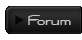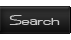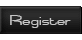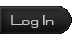PGM Studios :: JPOG :: JPOG Stuff :: Learn how to mod

# Dinosaur INI TutorialAuthorMessage
Dr.Thrax
Growing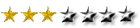Posts : 37
Join date : 2012-05-02
Age : 21
Location : Green LaboratorySubject: Dinosaur INI TutorialThu Jun 07, 2012 11:14 pm so here`s the TutorialWhat The Stats DoOkay. This is the actual tutorial! Allo, again, shall be used as a template. (Don't worry if your allo files are different, mine's modded a bit!)Unit {Name=allo;Directory=AUnitAllo; =what files it shall use for stuff like soundsTClass=AUnitCarcha; =what it behaves like?ScreenName=\$Unit_allo;=what the dino's name is ingameGUIDialog=AUnitDinosaurDialog;=??UICommand1=Orders;=This makes the Orders button appear when you select the dinoUICommand3=Species;=This makes the Species button appear when you select the dinoUICommand4=Info;=This makes the Info button appear when you select the dinoVOCreation=SFX_JP6J;=The VOCreation is the Voice to use for creation, which is SFX_JP6W:, which would be located in the music folder. If you were to get a sound file and rename it to SFX_JP6W:, that would be the sound file that is played instead of the lady announcing the dinos creationUseINX= 1 ;=??}Traits {Health=700.0;=How many "hit points" the dino hasVisual=75.0;=how well the dino can seeAudio=25.0;=how well the dino can hearSmell=50.0;=how well the dino can smellDamage=475.0;=how much damage an attack from the dino causesProtectMod=1.0;=??Territory=300.0;=how large the dino's territory isEnergy=250.0;=how long the dino can run without stopping to regain it's breathMetabolism=10.0;=how long a dino can go without eating?LifeSpan=8.5;=how long the dino can liveFoodVal=4.0;=if another dino (2) eats this dino, (1) how much nutrition dino 1 provides to 2.FoodReq=2.0;=how much food the dino requiresMass=4500.0;=how heavy it is? (It's ability to push other dinos around)Radius=2.0;=how wide the dino isCamRadius=10.0;=??VisRadius=10.0;=how wide the dino's visual radius isSpeed=11.0;=how fast the dino can runHeadRadius=0.8;=how wide the head is?MoveAnimSpeed=3.16;=how fast the dino's walking animations areRunAnimSpeed=8.04;=how fast the dino's running animations areMaxForce=15000.0;=??NHRate=2.0;=??GoalTolerance=4.0;=??Arrival=7.0;=how long you have to wait until the dinosaur is released from the hatchery?Acc=0.8;=how long it takes the dino to reach top speedDec=3.0;=how lond it takes for the dino to stopPathStrt=3.0;=??PlanStrt=3.0;=??FieldWt=3.0;=??TurnDamp=1.0;=how handicapped the dino's turning is?SteerLevel=1.0;=how fast the dino can turn?Size=50.5;=how large the dino "thinks" it is (have it up high enough, and the dino will be able to attack brachiosaurus!)BuildCost=0;=how much the building costs (For buildings)FenceBreak=3.0;=how high of a level fence the dino can breakFlockSize=4.0;=the maximum amount of dinos in a herd/packFlockCoh=10.0;=how much the subordinants follow the leader?FlockSep=5.0;=how far a dino has to go away from the flock until it is not a part of it anymoreFlockRad=20.0;=how far the heard will spread outCenterHeight=2.0;=??SelectionBoxRadius=4.0;=how big the selection box for the dino/building isLookH=2.36;=??LookV=1.57;=??StepCat=3.0;=??FleeMod=1.0;=??PredNeed=6.0;=how much prey the dino needs to kill before the hunting need goes awayPreyVal=6.0;=the value of killing a prey itemDecayTime=5.0;=how long it takes for the dead body to go awayRampageTime=5.0;=how long the dino rampagesComatoseTime=10.0;=how long the dino is in a coma for until it diesHungerThirstStress=0.001;=How much thirst and starving will stress the dinoTurnSpdThresh=0.1;=multiplies how fast it turns?}Flags {UnitFlags= 56625192 ;=??UnitFlagsID= 0 ;=??}Drives {Drive_Hunger=0.05;=how often the dino gets hungryDrive_Thirst=0.3;=how often the dino gets thirstyDrive_Defecate=0.0005;=how often the dino needs to poopDrive_Sleep=0.005;=how often it needs to sleepDrive_Defend=0.216;=how often the dino will defend itselfDrive_Play=0.216;=how often the dino will playDrive_Communicate=0.216;=how often the dino needs to communicate?}Animations {Model=allo;=what model the dino or building uses (I'd leave this alone if I were you)}PerceptionInputs {PInput_Organic=0.5;PInput_Repellent=0.0;PInput_Meat=1.0;PInput_Carnivore=1.0;)} these dictate if the dino is a herbivore or carnivorePInput_Herbivore=0.0;)PInput_Aquatic=0.0;=if the dino is aquatic (don't mess with this) PInput_Plant=0.0;=is it a plant?PInput_MaxSpeed=0.9;=a multiplier of how fast the dino can run?PInput_Size=2.0;=??PInput_Offensive=0.8;=how good the dino is at attacking?}PerceptionOutputs {POutput_Large_Carnivore_Food=0.0;=how often the dino class it describes will hunt the dino you are modding?POutput_Large_Carnivore_Drink=0.0;=if the dino class this describes can drink it?POutput_Large_Carnivore_Social=0.0;=if the dino will socialize with the dino class this describesPOutput_Large_Carnivore_Threat=0.0;=how much this dino class will threaten the dino you mod?POutput_Large_Carnivore_Competitor=9.0;=how much the dino compees with this dino classPOutput_Medium_Carnivore_Food=1.31;POutput_Medium_Carnivore_Drink=0.0;POutput_Medium_Carnivore_Social=0.0;POutput_Medium_Carnivore_Threat=0.0;POutput_Medium_Carnivore_Competitor=0.2;POutput_Small_Carnivore_Food=1.0;POutput_Small_Carnivore_Drink=0.0;POutput_Small_Carnivore_Social=0.0;POutput_Small_Carnivore_Threat=0.0;POutput_Small_Carnivore_Competitor=0.0;POutput_Large_Herbivore_Food=0.0;POutput_Large_Herbivore_Drink=0.0;POutput_Large_Herbivore_Social=0.0;POutput_Large_Herbivore_Threat=0.0;POutput_Large_Herbivore_Competitor=0.0;POutput_Medium_Herbivore_Food=0.8;POutput_Medium_Herbivore_Drink=0.0;POutput_Medium_Herbivore_Social=0.0;POutput_Medium_Herbivore_Threat=0.0;POutput_Medium_Herbivore_Competitor=0.0;POutput_Small_Herbivore_Food=1.0;POutput_Small_Herbivore_Drink=0.0;POutput_Small_Herbivore_Social=0.0;POutput_Small_Herbivore_Threat=0.0;POutput_Small_Herbivore_Competitor=0.0;POutput_Human_Food=3.8;POutput_Human_Drink=0.0;POutput_Human_Social=0.0;POutput_Human_Threat=0.0;POutput_Human_Competitor=0.0;POutput_Plant_Food=0.0;POutput_Plant_Drink=0.0;POutput_Plant_Social=0.0;POutput_Plant_Threat=0.0;POutput_Plant_Competitor=0.0;POutput_Vehicle_Food=0.0;POutput_Vehicle_Drink=0.0;POutput_Vehicle_Social=0.0;POutput_Vehicle_Threat=0.5;POutput_Vehicle_Competitor=0.0;POutput_Repellent_Object_Food=0.0;POutput_Repellent_Object_Drink=0.0;POutput_Repellent_Object_Social=0.0;POutput_Repellent_Object_Threat=1.0;POutput_Repellent_Object_Competitor=0.0;}DinoInfo {Rarity= 2 ;=??Flags= 1 ;=??Period= 1 ;=??Worth= 4 ;=the dinosaurs stars or rating.Loc1= 0 ;=??Loc2= 100 ;=??Loc3= 0 ;=??Loc4= 0 ;=??Batch= 1 ;=??Class= 3 ;=??}UnitSpecific {}DNATraits {Aggression=9.0;=how aggressive the dino isPlayfulness=0.0;=how playful the dino isAppetite=0.0;=how big the dino's appetite isEnergy=0.0;=??Speed=0.0;=??LethalGene=-1.0;=dictates whether you can activate LethalGene on the dino}Dinosaur INI TutorialPage 1 of 1
 Similar topics» Curl Tutorial!
» AOE_Fan's RMS Command Tutorial
» How to make .abk book ( Arena ) step by step... tutorial...
» i need help ;/
» IvanHoe 999946f x64 vs. IvanHoe B46fB x64, 2.800 games, still running

Permissions in this forum:You cannot reply to topics in this forumJump to: Select a forum||--Rules|   |--Rules|   |--JPOG|   |--Skins|   |   |--Environment Skins|   |   |--Not Ingame Skins|   |   |--Skin Requests|   |   |   |--Mods|   |   |--Sounds|   |   |   |--Expansion Packs|   |   |--In Progress EP|   |   |   |--JPOG Stuff|   |   |--Learn how to mod|   |   |   |--JPOG questions|   |--Mod/Skin junkyard|   |--Site|   |--Announcements|   |--Guests zone|   |--RPGs|   |--Library|   |--Science, Research and Prehistory|   |--Modern Science and Technology|   |--Dinosaur Discoveries|   |--Art Stuff|   |--Art Shop|   |--Unreal Art|   |--Horror Art|   |--Environment Art|   |--Animal Art|   |--Anatomy Art|   |--Creations